# 13+ Simple Algebra Worksheet Templates -Word, PDF

Algebra is one of the most crucial aspects of mathematics. If you are a maths teacher, you must make sure to get your students enough practice of the algebra problems to strengthen their algebra aptitude. The best way to ensure good algebra practice is to go for Simple algebra worksheets for the beginners. Now, creating a complete algebra worksheet every now and then for all students in your class is obviously a daunting affair.

## Algebra Worksheets For Beginners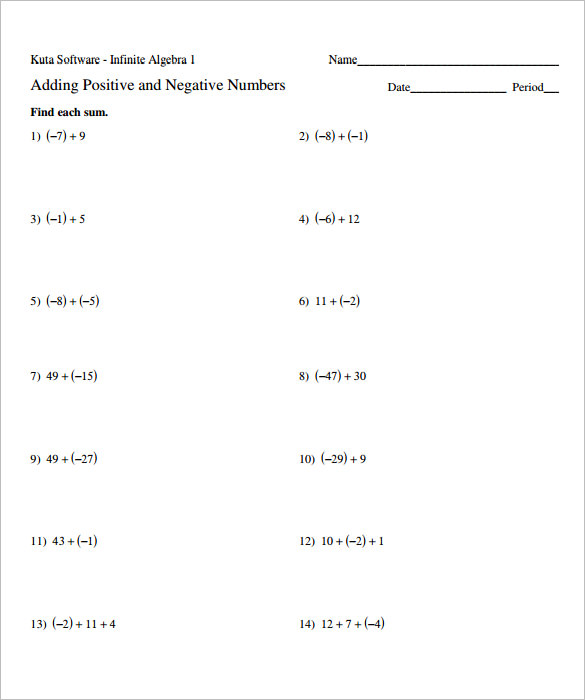## Algebraic Expressions Worksheets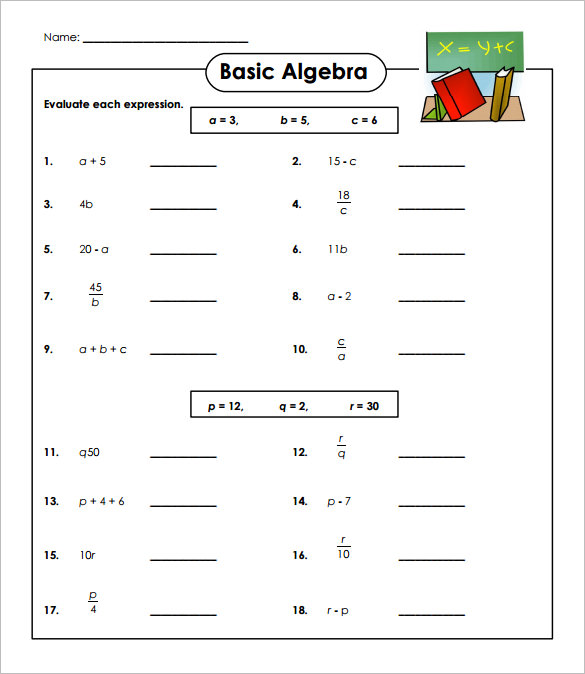## Basic Algebra Work Sheet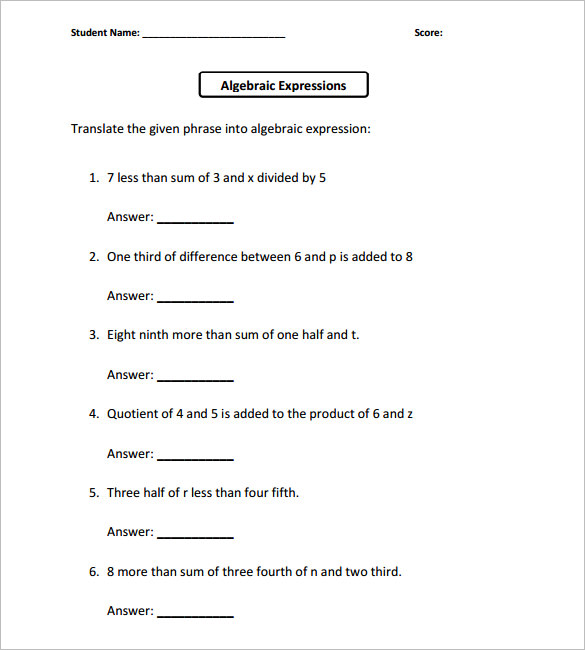## Free Algebra Worksheet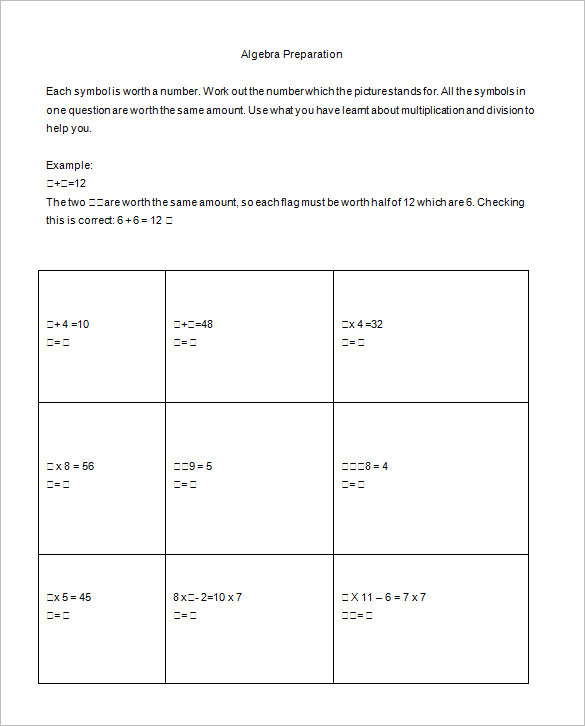## Pre Algebra Worksheet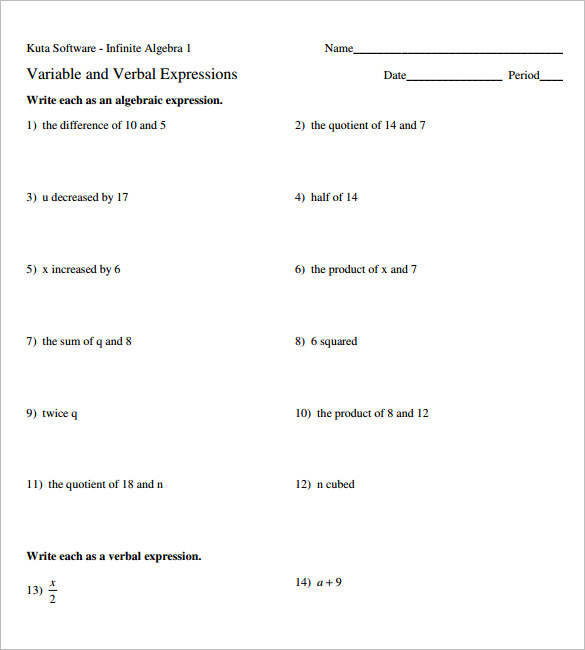## Printable Algebra Worksheet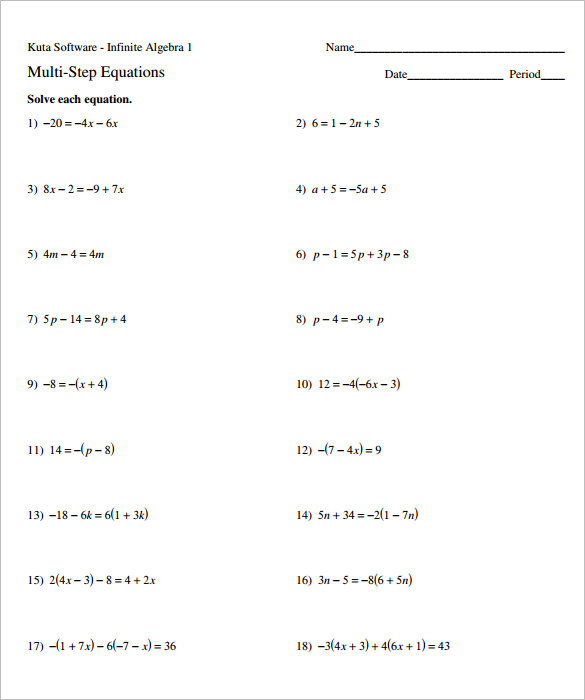## Simple Algebra Equations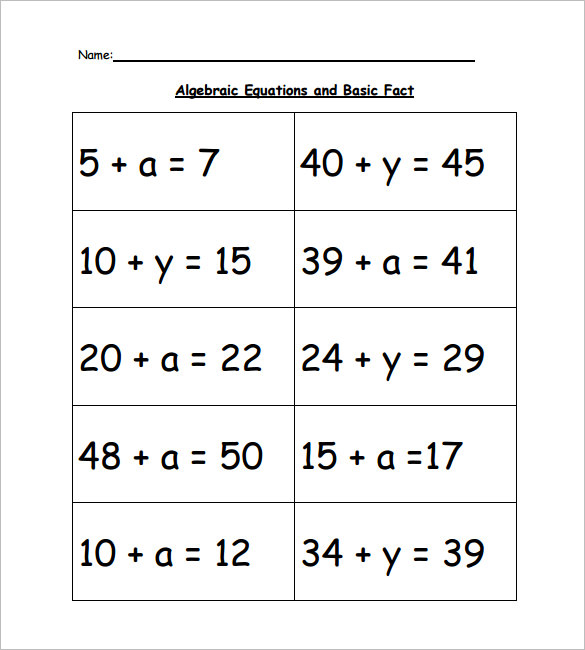## Simple Algebra Problems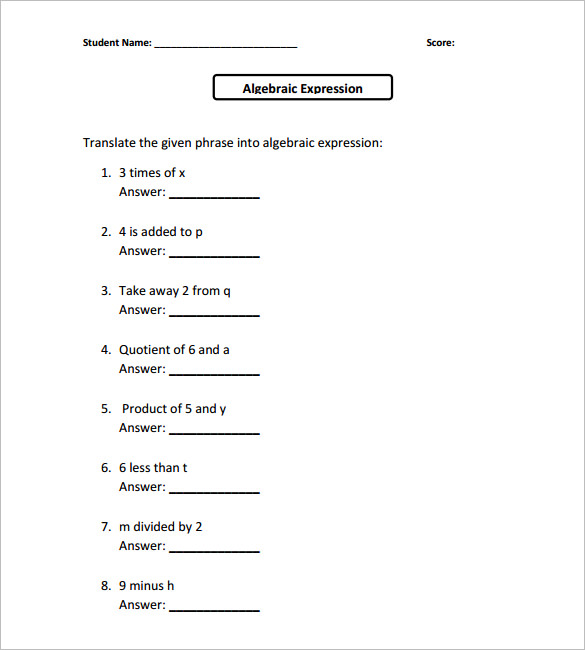## 7th Grade Algebra Practice Problems## Simple Algebra Questions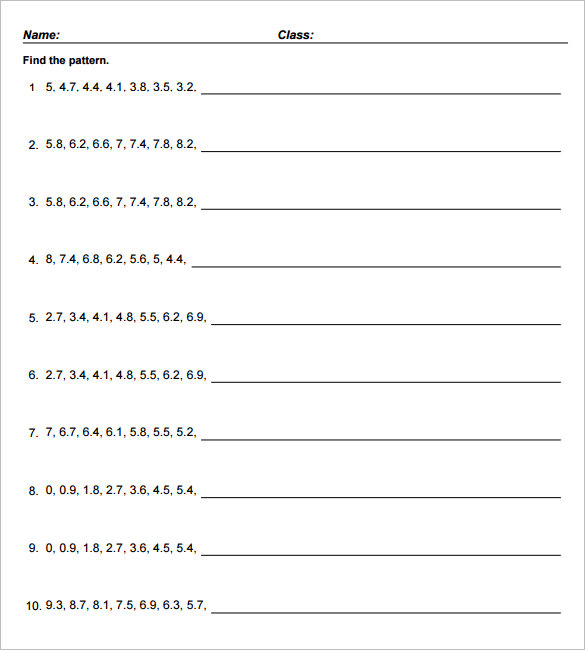## Sample 7th Grade Algebra Examples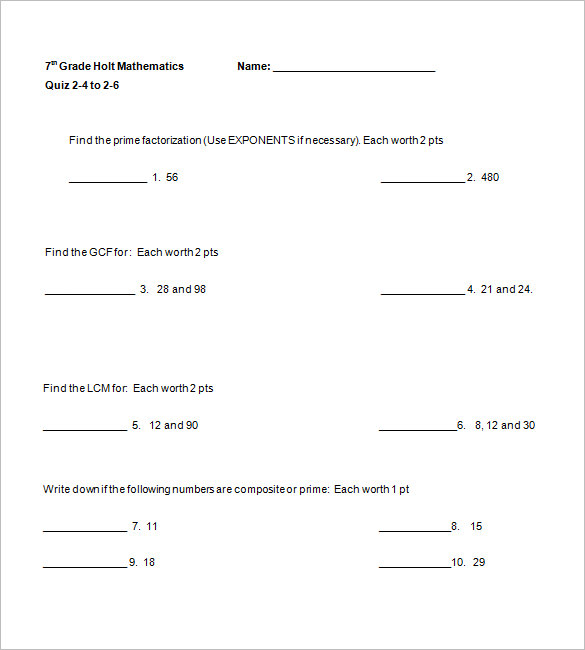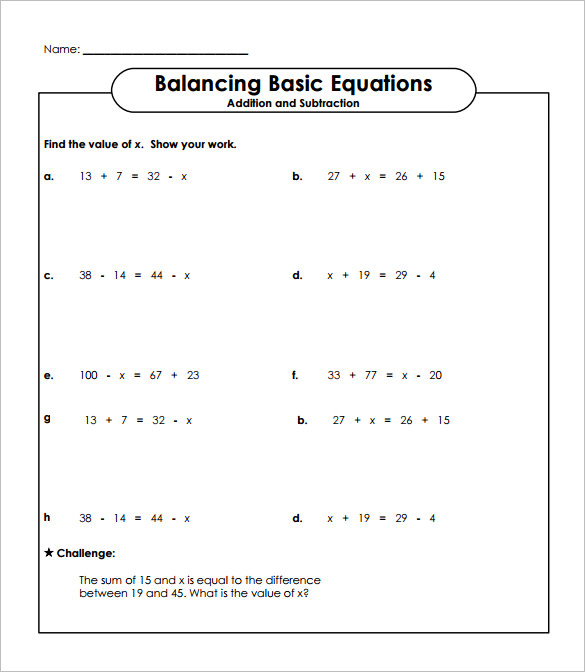## Sample Simple Equations Worksheet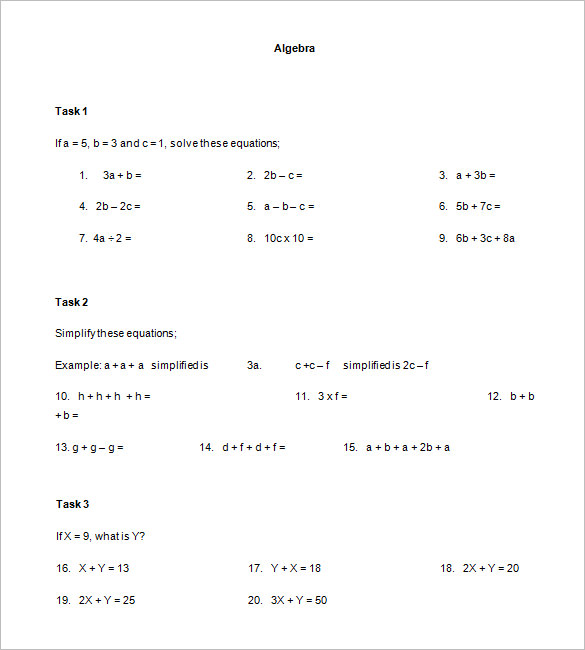But not to worry any more as these days you are getting sample Simple algebra worksheets templates over the internet.

## Brief on Simple algebra worksheets online

There are several online sites that offer free Simple algebra worksheets with pre-structured algebra sums so that the teacher does not need to create the worksheet from scratch. You would simply need to pass these on to the students for calculation.

• These templates are easily accessible online, saving you great deal of time and energy.
• These online worksheets could be used for school students while you will also get simple college algebra worksheets for college students as well.
• You can use the same worksheet for every student in your class.